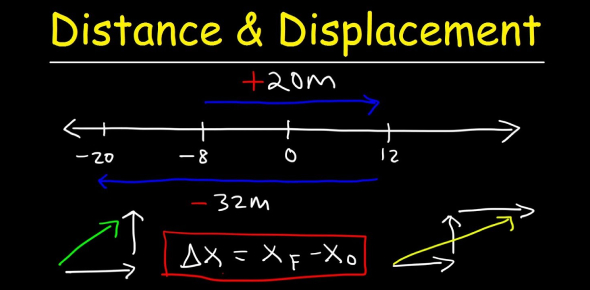# Distance And Displacement Quiz

10 Questions | Attempts: 13157SettingsDistance and displacement are two very important topics in Physics. Today we're going to test your knowledge with this distance and displacement quiz. When you move an object from its original position using some force, one can project just how far it can go given its weight. The quiz below will help you understand just how much you understood about distance and displacement and the factors that affect just how far an object will move. Take it up and see how much you score.

• 1.
A softball player leaves the batter's box, overruns first base by 3.0 meters, and then returns to first base. Compared to the total distance traveled by the player, the magnitude of the player's total displacement from the batter's box is
• A.

Smaller

• B.

Larger

• C.

The same

• 2.
What is the total displacement of a student who walks 3 blocks east, 2 blocks north, 1 block west, and then 2 blocks south?
• A.

2 blocks east

• B.

2 blocks west

• C.

8 blocks

• D.

0

• 3.
A girl leaves a history classroom and walks 10. meters north to a drinking fountain. Then she turns and walks 30. meters south to an art classroom. What is the girl's total displacement from the history classroom to the art classroom?
• A.

20. m south

• B.

20. m north

• C.

40. m north

• D.

40. m south

• 4.
What is the SI unit for displacement?
• A.

Centimetre

• B.

Metre

• C.

Kilometre

• D.

None of the above

• 5.
Distance is a ______ quantity.
• A.

Scalar

• B.

Vector

• C.

Negative

• D.

Positive

• 6.
A car travels 20. meters east in 1.0 second. The displacement of the car at the end of this 1.0-second interval is
• A.

20. m/s

• B.

20. m

• C.

20. m east

• D.

20. m/s east

• 7.
A student walks 1.0 kilometer due east and 1.0 kilometer due south. Then she runs 2.0  kilometers due west. The magnitude of the student's resultant displacement is closestto
• A.

3.4 km

• B.

1.4 km

• C.

4.0 km

• D.

0 km

• 8.
If an object, after traveling a certain distance returns to the starting point, what will be its displacement?
• A.

One

• B.

Zero

• C.

More than one

• D.

Less than one

• 9.
John, an athlete covers 3 rounds on a circular track of radius 50 m. What is the displacement covered by him?
• A.

Zero

• B.

4 m

• C.

12 m

• D.

50 m

• 10.
Michael travels 250 miles to North but then back-tracks to South for 105 miles to pick up a friend. Calculate Michael's total displacement.
• A.

189 mi N

• B.

145 mi N

• C.

145 mi S

• D.

114 mi S

## Related TopicsBack to top
×

Wait!
Here's an interesting quiz for you.